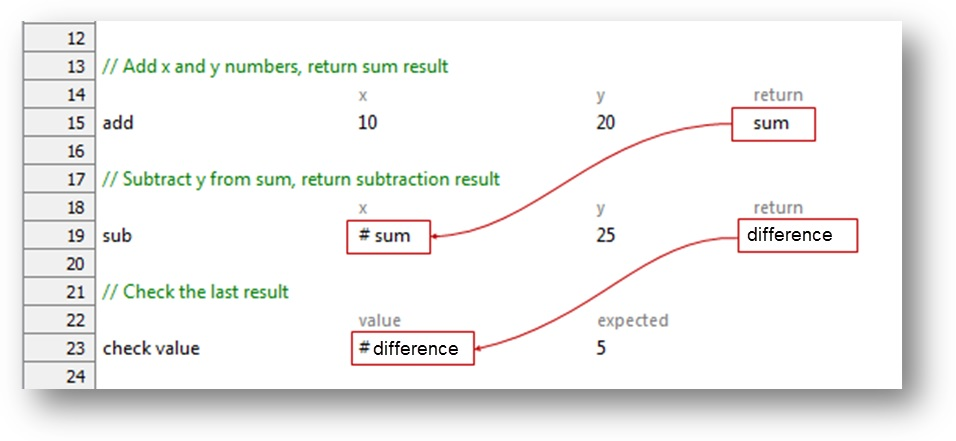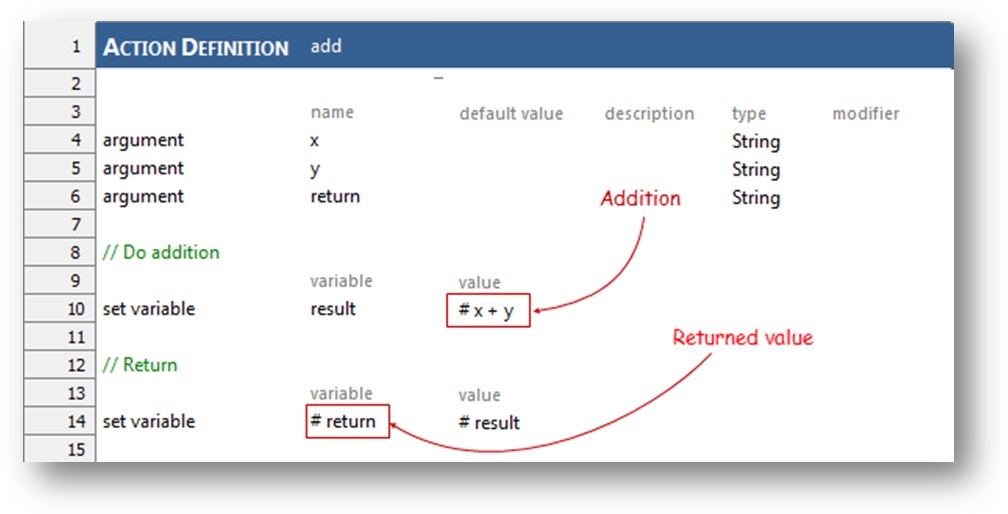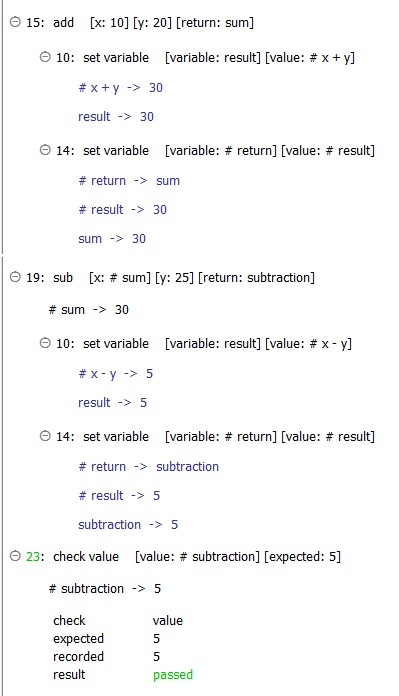# User cases

In some test scenarios, you may want to create a user-defined action that, after doing a bunch of stuff, returns one or more values to the calling action or test case. The caller may then use the returned value(s) at a later stage of execution. The question is, “How do I return values from an action?”

To use a simplified example, say we need to define an action, add, to perform an addition operation on two variables, then return the result to the test. In the calling action, the returned result is assigned to a variable, sum, to then be submitted to another action, sub, to have a value subtracted from it. The returned subtraction result, assigned to the variable difference, is in turn used for a comparison.# Solutions

To return a value, a user-defined action needs to create an argument to store it. In the example below, we define the add action, which declares the argument return, which is the means by which the result of the addition operation is to be returned. After the addition operation, which stores the sum in the variable result, a set variable action is used to transfer that sum to the return argument.

Note that the expression symbol ‘#’ precedes return in the variable argument of the second set variable action (line 14), while no such symbol is required in the first set variable action. That’s because set variable expects a normal variable in that argument, which it gets in the first case (line 10). In the second case (line 14), we are informing TestArchitect that, rather than set the value to the argument return, we want it assigned to the variable from the calling action which return represents. (That variable in the caller is sum.)

# Examples

Test lines:The definition of the user-defined sub action works in a similar manner:Result: